## Molecular Shapes and Polarity

### Learning Objective

1. Determine the shape of simple molecules.
2. Determine the polarity of molecules using net molecular dipoles.

Molecules have shapes. There is an abundance of experimental evidence to that effect—from their physical properties to their chemical reactivity. Small molecules—molecules with a single central atom—have shapes that can be easily predicted.

The basic idea in molecular shapes is called valence shell electron pair repulsion (VSEPR). It says that electron pairs, being composed of negatively charged particles, repel each other to get as far away from each other as possible. VSEPR makes a distinction between electron group geometry, which expresses how electron groups (bonding and nonbonding electron pairs) are arranged, and molecular geometry, which expresses how the atoms in a molecule are arranged. However, the two geometries are related.

There are two types of electron groups: any type of bond—single, double, or triple—and lone electron pairs. When applying VSEPR to simple molecules, the first thing to do is to count the number of electron groups around the central atom. Remember that a multiple bond counts as only one electron group.

Any molecule with only two atoms is linear. A molecule whose central atom contains only two electron groups orients those two groups as far apart from each other as possible—180° apart. When the two electron groups are 180° apart, the atoms attached to those electron groups are also 180° apart, so the overall molecular shape is linear. Examples include BeH2 and CO2:A molecule with three electron groups orients the three groups as far apart as possible. They adopt the positions of an equilateral triangle—120° apart and in a plane. The shape of such molecules is trigonal planar. An example is BF3:Some substances have a trigonal planar electron group distribution but have atoms bonded to only two of the three electron groups. An example is GeF2:From an electron-group-geometry perspective, GeF2 has a trigonal planar shape, but its real shape is dictated by the positions of the atoms. This shape is called bent or angular.

A molecule with four electron groups around the central atom orients the four groups in the direction of a tetrahedron, as shown in Figure 9.4 “Tetrahedral Geometry.” If there are four atoms attached to these electron groups, then the molecular shape is also tetrahedral. Methane (CH4) is an example.

This diagram of CH4 illustrates the standard convention of displaying a three-dimensional molecule on a two-dimensional surface. The straight lines are in the plane of the page, the solid wedged line is coming out of the plane toward the reader, and the dashed wedged line is going out of the plane away from the reader.

NH3 is an example of a molecule whose central atom has four electron groups but only three of them are bonded to surrounding atoms.Although the electron groups are oriented in the shape of a tetrahedron, from a molecular geometry perspective, the shape of NH3 is trigonal pyramidal.

H2O is an example of a molecule with a central atom that has four electron groups but only two of them are bonded to surrounding atoms.Although the electron groups are oriented in the shape of a tetrahedron, the shape of the molecule is bent or angular. A molecule with four electron groups around the central atom but only one electron group bonded to another atom is linear because there are only two atoms in the molecule.

Double or triple bonds count as a single electron group. CH2O has the following Lewis electron dot diagram.The central C atom has three electron groups around it because the double bond counts as one electron group. The three electron groups repel each other to adopt a trigonal planar shape:(The lone electron pairs on the O atom are omitted for clarity.) The molecule will not be a perfect equilateral triangle because the C–O double bond is different from the two C–H bonds, but both planar and triangular describe the appropriate approximate shape of this molecule.

### Example 10

What is the approximate shape of each molecule?

1. PCl3
2. NOF

Solution

The first step is to draw the Lewis electron dot diagram of the molecule.

1. For PCl3, the Lewis electron dot diagram is as follows:The lone electron pairs on the Cl atoms are omitted for clarity. The P atom has four electron groups with three of them bonded to surrounding atoms, so the molecular shape is trigonal pyramidal.

2. The Lewis diagram for NOF is as follows:The N atom has three electron groups on it, two of which are bonded to other atoms. The molecular shape is bent.

Test Yourself

What is the approximate molecular shape of CH2Cl2?

Tetrahedral

Table 9.4 “Summary of Molecular Shapes” summarizes the shapes of molecules based on their number of electron groups and surrounding atoms.

Table 9.4 Summary of Molecular Shapes

Number of Electron Groups on Central Atom Number of Surrounding Atoms Molecular Shape
any 1 linear
2 2 linear
3 3 trigonal planar
3 2 bent
4 4 tetrahedral
4 3 trigonal pyramidal
4 2 bent

## Molecular Polarity

The overall polarity of molecules with more than one bond is determined from both the polarity of the individual bonds and the shape of the molecule. Each bond’s dipole moment can be treated as a vector quantity, having a magnitude and direction. Therefore the molecular polarity is the vector sum of the individual bond dipoles.

### How to determine the vector sum

One method to determine the vector sum of dipole arrows is known as the tail-to-head method. Let’s examine this method for a molecule of water. 1. First draw the Lewis electron dot diagram for water and determine its molecular shape. Water has four electron groups, but only two atoms attached to the central atom so it is bent.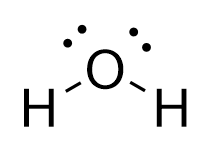2. Draw in dipole arrows for all polar covalent bonds, starting the arrow at the more electropositive atom, and ending at the more electronegative atom.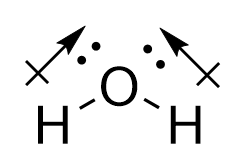3. Connect the dipole arrows tail-to-head.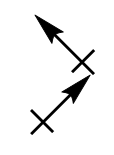4. Draw a new line connecting the tail of the first vector. This is the net molecular dipole.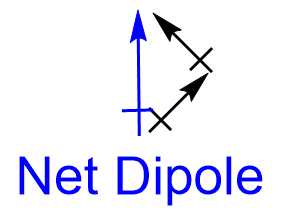5. Now superimpose the net molecular dipole arrow onto the molecule.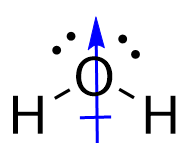An alternative method to determine the vector sum of dipole arrows is known as the vector component method. Let’s examine this method again for a molecule of water. The first two steps remain the same as the tail-to-head method: 1. First draw the Lewis electron dot diagram for water and determine its molecular shape. Water has four electron groups, but only two atoms attached to the central atom so it is bent.

2. Draw in dipole arrows for all polar covalent bonds, starting the arrow at the more electropositive atom, and ending at the more electronegative atom.

3. For dipole arrows at an angle, separate them into horizontal and vertical vector components.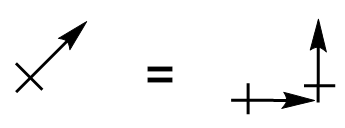4. Superimpose the vector components onto the molecule.
5. Cancel out any vector components that are of equal magnitude and pointing in the opposite direction.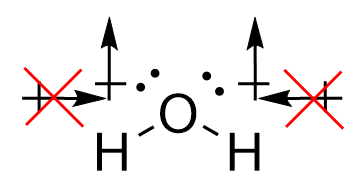As a result of the vector quantity nature of bond dipoles, some molecules may contain polar bonds, yet have no net molecular dipole moment. For example, CO2:

### Key Takeaways

• The approximate shape of a molecule can be predicted from the number of electron groups and the number of surrounding atoms.
• The molecular polarity can be established by determining the vector sum of all bond dipoles.

### Exercises

1. What is the basic premise behind VSEPR?

2. What is the difference between electron group geometry and molecular geometry?

3. Identify the electron group geometry and molecular geometry of each molecule.

a)  H2S

b)  POCl3

4.  Identify the electron group geometry and the molecular geometry of each molecule.

a)  CS2

b)  H2S

5.  Identify the electron group geometry and the molecular geometry of each molecule.

a)  HCN

b)  CCl4

6.  Identify the electron group geometry and the molecular geometry of each molecule.

a)  BI3

b)  PH3

7.  What is the geometry of each species?

a)  CN

b)  PO43−

8.  What is the geometry of each species?

a)  PO33−

b)  NO3

9.  What is the geometry of each species?

a)  COF2

b)  C2Cl2 (both C atoms are central atoms and are bonded to each other)

10.  What is the geometry of each species?

a)  CO32−

b)  N2H4 (both N atoms are central atoms and are bonded to each other)

11. Determine the net molecular dipole of each species:

a) BF3

b) NH3

1. Electron pairs repel each other.

3.

a) electron group geometry: tetrahedral; molecular geometry: bent

b) electron group geometry: tetrahedral; molecular geometry: tetrahedral

5.

a) electron group geometry: linear; molecular geometry: linear

b) electron group geometry: tetrahedral; molecular geometry: tetrahedral

7.

a) linear

b) tetrahedral

9.

a) trigonal planar

b) linear and linear about each central atom

11.